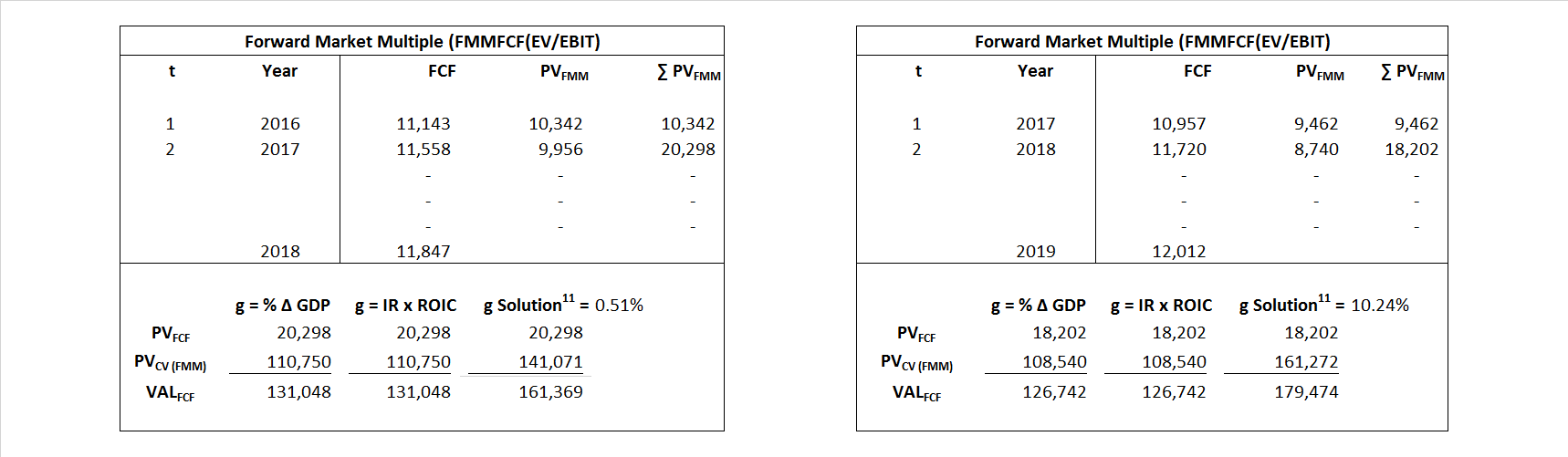# Intel

## Analyst Listing

The following analysts provide coverage for the subject firm as of May 2016:

 Broker Analyst Analyst Email BMO Capital Markets Ambrish Srivastava ambrish.srivastava@bmo.com RBC Capital Markets Amit Daryanani amit.daryanani@rbccm.com Aegis / HIRG Technology Ashok Kumar akumar@aegiscap.com Wedbush Securities Betsy Van Hees betsy.vanhees@wedbush.com Evercore ISI C.J. Muse cj.muse@evercoreisi.com B Riley & Co Craig A. Ellis cellis@brileyco.com Wells Fargo Securities David Wong david.m.wong@wellsfargo.com CRT Capital Group Douglas Freedman dfreedman@sterneageecrt.com Raymond James Hans Mosesmann hans.mosesmann@raymondjames.com Credit Suisse John Pitzer john.pitzer@credit-suisse.com Stifel Nicolaus Kevin E Cassidy kcassidy@stifel.com Roth Capital Partners Krishna Shankar kshankar@roth.com Jefferies Mark Lipacis mlipacis@jefferies.com Canaccord Genuity Matthew D. Ramsay mramsay@canaccordgenuity.com Pacific Crest Securities-KBCM Michael McConnell mmcconnell@pacific-crest.com Brean Capital, LLC Mike Burton mburton@breancapital.com Oppenheimer Rick Schafer rick.schafer@opco.com Nomura Research Romit Shah romit.shah@nomura.com Deutsche Bank Research Ross Seymore ross.seymore@db.com Bernstein Research Stacy A. Rasgon stacy.rasgon@bernstein.com Topeka Capital Markets Suji De Silva sd@topekacapitalmarkets.com Northland Securities Thomas Sepenzis tsepenzis@northlandcapitalmarkets.com Cowen & Company Timothy Arcuri timothy.arcuri@cowen.com Mizuho Securities USA Vijay Rakesh vijay.rakesh@us.mizuho-sc.com SunTrust Robinson Humphrey William Stein william.stein@suntrust.com Needham Y. Edwin Mok emok@needhamco.com Daiwa Securities Co. Ltd. Yoko Yamada yoko.yamada@us.daiwacm.com

## Primary Input Data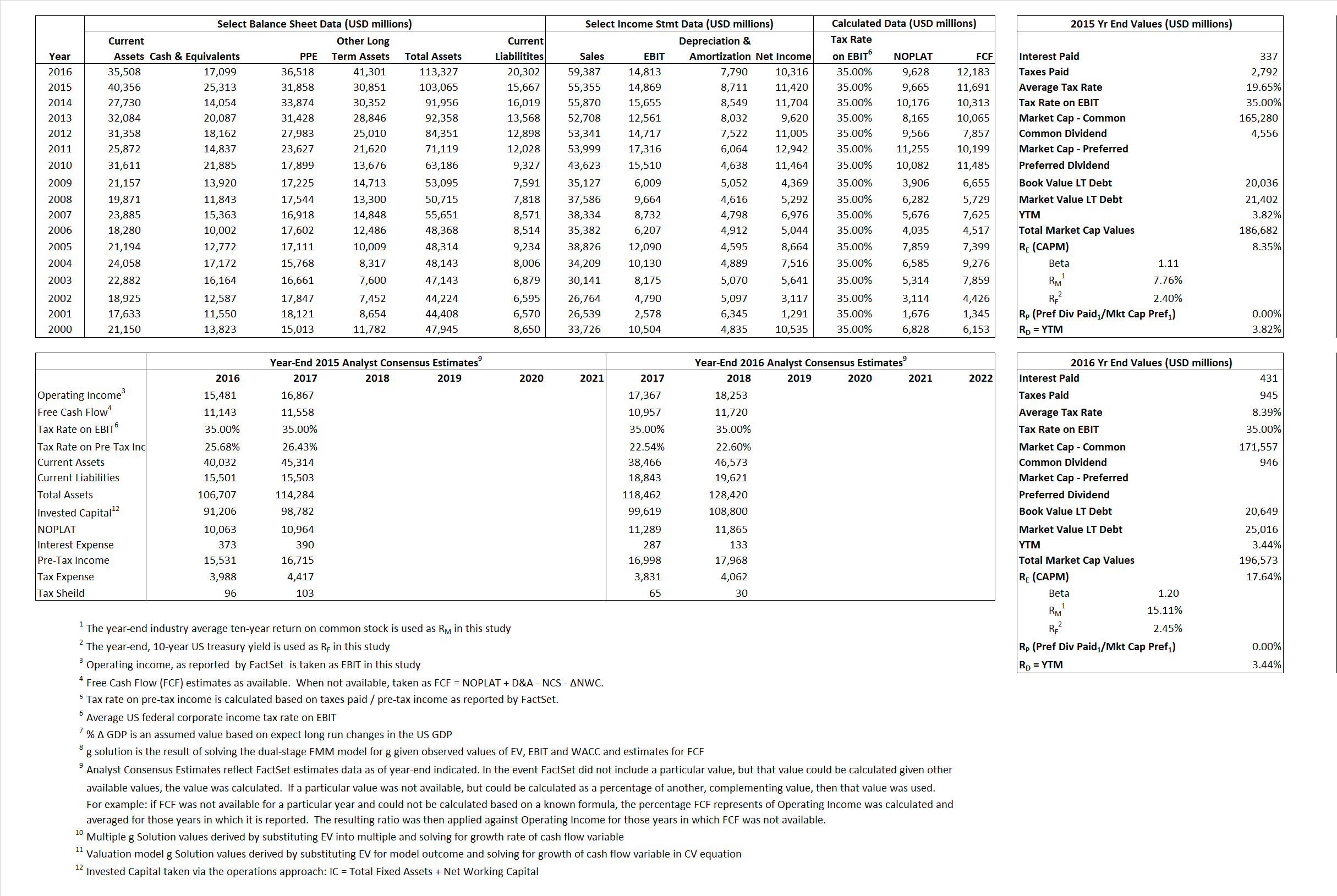## Derived Input Data

### Equational Form

Net Operating Profit Less Adjusted Taxes NOPLAT   9,665  9,628$NOPLAT\, =\, EBIT\, x\, (1 \,-\, Avg \,\,Tax\,\, Rate\,\, on\,\, EBIT)$
Free Cash Flow FCF  11,691  12,183$FCF\,=NOPLAT\,+\,Non-Cash\,Expenses-\Delta NWC\,-\,NCS$
Tax Shield TS  66  36$TS\,=\,Interest\,\,Paid\,\,x\,\, Avg \,\,Tax\,\,Rate\,\, on\,\, Pre-Tax\,\, Income$
Invested Capital IC  87,398  93,025$IC\,=\,Fixed\,\,Operating\,\,Assets\,\,+\,\,Net\,\, Working\,\, Capital$
Return on Invested Capital ROIC 11.06% 10.35%$ROIC\,=\,\frac { NOPLAT }{ IC }$
Net Investment NetInv  20,172  13,417$NetInv\,=\,{ {IC}_{1}}-{{IC}_{0}}+Depreciation$
Investment Rate IR 208.72% 139.35%$IR\,=\,\frac {NetInv}{NOPLAT}$
Weighted Average Cost of Capital WACCMarket 7.74% 15.80%$WACC\,=\,\frac { E }{ V } { R }_{ E }\,+\,\frac { P }{ V } { R }_{ P }\,+\,\frac { D }{ V } { R }_{ D }\left( 1- Avg\,\, Tax\,\,Rate\,\,on\,\,Pre-Tax\,\,Income \right)$
WACCBook  9.18% 9.22%
Enterprise value EVMarket  161,369  179,474$EV\,=\,Market\,\,Cap\,\,Equity\,+\,\,Long\,\,Term\,\,Debt\,-\,Cash$
EVBook  168,217  175,107
EV/EBIT Multiple$\frac{EV_{Market}}{EBIT}$  10.85  12.12$EV/EBIT\,=\,\frac { EV}{ EBIT}$
Long-Run Growth g = IR x ROIC
23.08%   14.42% Long-run growth rates of the income variable  are used in the Continuing Value portion of the valuation models.
g = %$\Delta$ GDP   2.50%   2.50%

## Valuation Model Outcomes

The outcomes presented in this study are the result of original input data, derived data, and synthesized inputs and, depending on the equational form of any particular valuation model, may result in irrelevant or implausible results.  For example, in the event WACC < g, the value of this term, often found in the denominator of an equation’s continuation value term, will be expressly negative and may result in a negative overall valuation for the firm.  In the event of a WACC < g relation, the model form as applied to the subject firm offers an irrelevant outcome.

### Equational form

Key Value Driver (NOPLAT) KVD (NOPLAT)${ Value }_{ DCF/KVD }=\sum { \frac { NOPLAT_{ t } }{ { \left( 1+WACC \right) }^{ t } } +\frac { \frac { { NOPLAT }_{ 1 }\left( 1-\frac { g }{ ROIC } \right) }{ WACC-g } }{ { \left( 1+WACC \right) }^{ t } } }$Key Value Driver (FCF) KVD (FCF)${ Value }_{ DCF/KVD }=\sum { \frac { FCF_{ t } }{ { \left( 1+WACC \right) }^{ t } } +\frac { \frac { { NOPLAT }_{ 1 }\left( 1-\frac { g }{ ROIC } \right) }{ WACC-g } }{ { \left( 1+WACC \right) }^{ t } } }$Free Cash Flow FCF${ Value }_{ DCF/FCF }=\sum { \frac { FCF_{ t } }{ { \left( 1+WACC \right) }^{ t } } +\frac { \frac { { FCF }_{ 1 }}{ WACC-g } }{ { \left( 1+WACC \right) }^{ t } } }$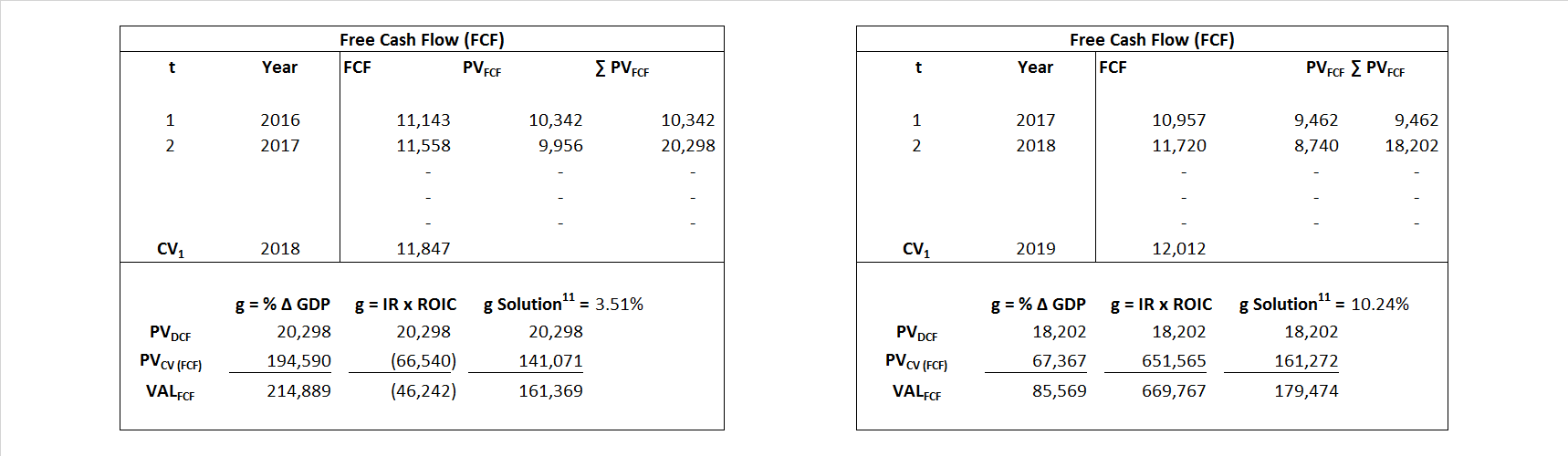Economic Profit ECON π${ Value }_{ { ECON\pi } }= I{ C }_{ 0 }+\sum { \frac { { IC }_{ t-1 }(ROI{ C }_{t}-WAC{C}_{t}) }{ { \left( 1+WACC \right) }^{ t } }+ \frac {\frac { I{C}_{0}\ x\ (ROI{C}_{1}\ -\ WAC{C}_{1}) }{ WACC-g } }{ { \left( 1+WACC \right) }^{ t } } }$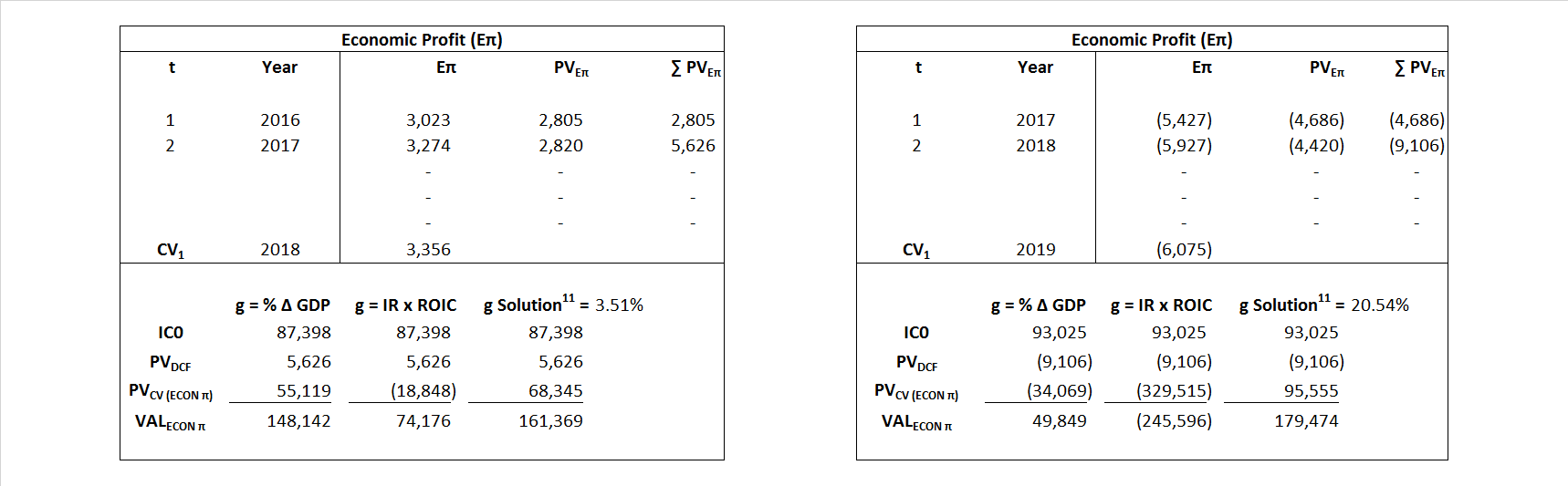Adjusted Present Value APV${ Value }_{ APV }=\sum { \frac { FCF_{ t } }{ { \left( 1+{ k }_{ u } \right) }^{ t } } +\frac { \frac { { FCF }_{ 1 }}{ { k }_{ u }-g } }{ { \left( 1+{ k }_{ u } \right) }^{ t } } } +\sum { \frac { { TS }_{ t } }{ { \left( 1+{ k }_{ tax } \right) }^{ t } } +\frac { \frac { { TS }_{ 1 }}{ { k }_{ tax }-g } }{ { \left( 1+{ k }_{ tax } \right) }^{ t } } }$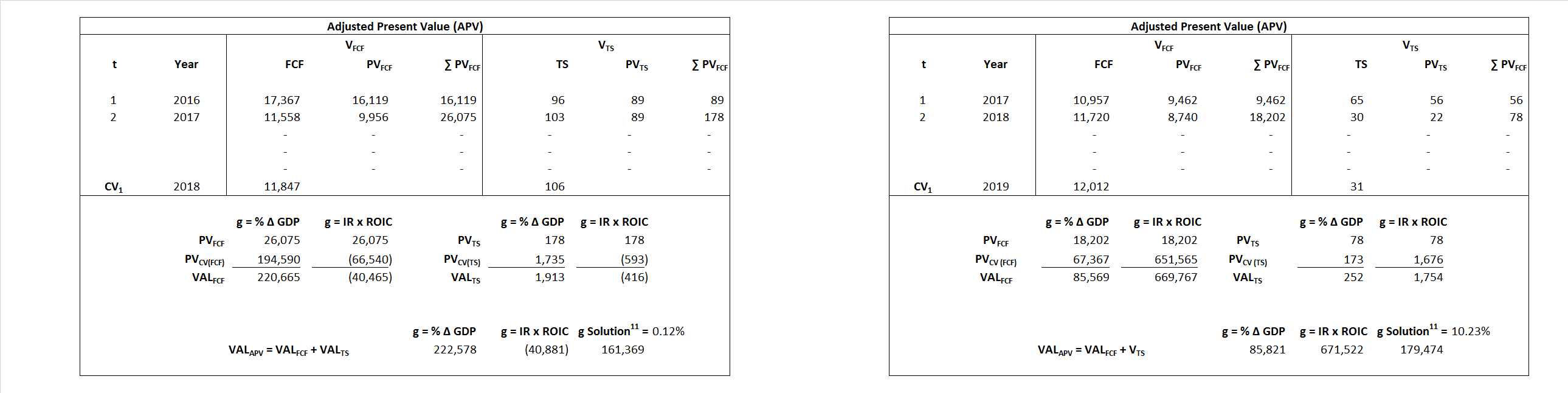Forward Market Multiple FMM${ Value }_{ DCF/FMM}=\sum { \frac { FCF_{ t } }{ { \left( 1+WACC \right) }^{ t } } +\frac { { EBIT }_{ 1 }\,{x}\,{FMM}}{ { \left( 1+WACC \right) }^{ t } } }{\,\,\,; \,\,FMM\,=\,\frac{{EV}_{t=0}}{{EBIT}_{t=0}}}$